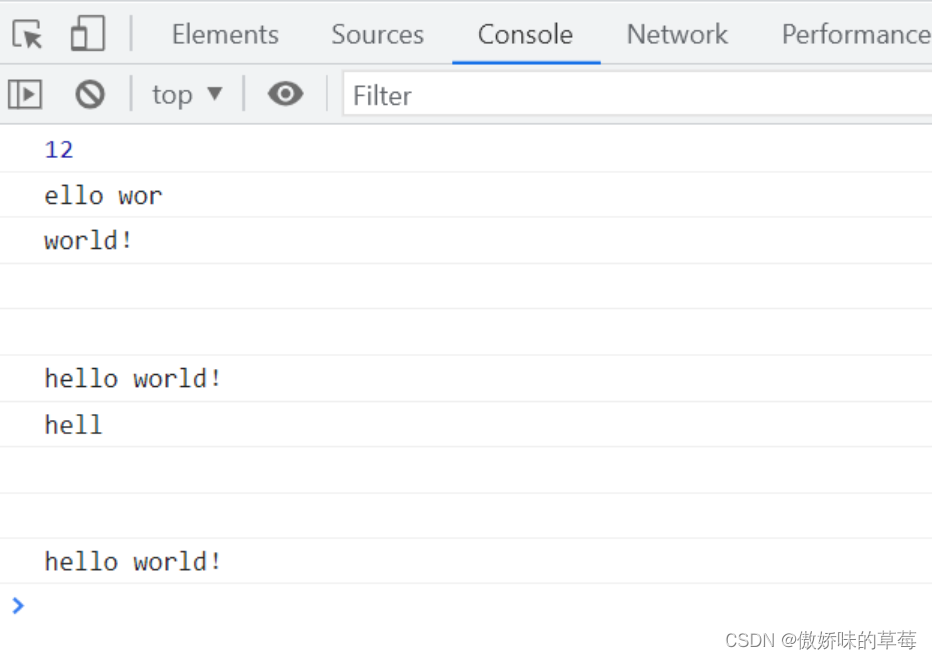# js字符串分割處理的幾種方法(6種)

## 1、slice(start, end)

```    let test = 'hello world！'
console.log(test.length)

console.log(test.slice(1, 9))
console.log(test.slice(6))
console.log(test.slice(9, 1))
console.log(test.slice(-2))
console.log(test.slice(0, -2))
console.log(test.slice(-4, -2))
console.log(test.slice(-2, 4))
```①第一個參數比第二個參數大，結果返回空字符串

②傳入參數是負數，slice()會先做運算 test.length + 負數參數。

## 2、substr(start, length)

```    let test = 'hello world！'
console.log(test.length)

console.log(test.substr(1, 9))
console.log(test.substr(6))
console.log(test.substr(9, 9))
console.log(test.substr(20))
console.log(test.substr(-2))
console.log(test.substr(-8, 4))
console.log(test.substr(-8, 0))
console.log(test.substr(-8, -4))
console.log(test.substr(-20))
```①傳入參數超過length返回空字符串

②傳入負數，則從字符串的尾部開始算起始位置，-1指最後一個字符，-2指倒數第二個字符；當傳入的第一個參數是負數且它的絕對值超過length，這個負數轉化為0，當傳入的第二個參數是負數，等價於0，截取0個字符，返回空字符串。

## 3、substring(start, stop)

```    let test = 'hello world！'
console.log(test.length)

console.log(test.substring(1, 9))
console.log(test.substring(6))
console.log(test.substring(9, 9))
console.log(test.substring(20))
console.log(test.substring(-2))
console.log(test.substring(-8, 4))
console.log(test.substring(-8, 0))
console.log(test.substring(-8, -4))
console.log(test.substring(-20))
```①第二個參數==第一個參數，返回空字符串

②傳入兩個參數，不管在第一還是第二位置，都會將小的參數作為第一個參數，較大的作為第二個參數

③任何一個參數為負數或者NaN的時候，自動將其轉換為0

④任何一個參數大於length，按照length處理

js字符串截取三姐妹，都不會對原始的字符串進行修改，而是返回新的子集。但是三姐妹各自有各自的個性，面對同一種參數處理的方式都是不一樣的。

## 4、split(separator, length)

```//以空格為分隔符輸出數組
var str = '123 abc 1 2 3 a b c '
var arr = str.split(' ')
console.log(arr)
``````    var str = '123 abc 1 2 3 a b c'
var arr = str.split(' ', 4)
//第二個參數表示返回數組的最大長度！註意不是原來字符串的，是新輸出的數組的
console.log(arr)
```## 5、join(separator)

```    var a = ['I', 'am', 'a', 'girl', '英文名', '是', 'gaby']
var arr = a.join(',')
console.log(arr)
```## 6、splice(start, length, …args)

```//刪，第一個參數為第一項位置，第二個參數為要刪除幾個 0數起
//array.splice(index,num)，返回值為刪除內容，array為結果值
var arr = ['a', 'b', 'c', 'd', 'e', 'f']
console.log(arr.splice(0, 4))
console.log(arr)
``````//增，第一個參數（插入位置），第二個參數（0），第三個參數（插入的項）
//array.splice(index,0,insertValue)，返回值為空數組，array值為最終結果值
var arr = ['a', 'b', 'c', 'd', 'e', 'f']
console.log(arr.splice(2, 0, 'insert'))
console.log(arr)
``````//改 第一個參數（起始位置），第二個參數（刪除的項數），第三個參數（插入任意數量的項）
//array.splice(index,num,insertValue)，返回值為刪除內容，array為結果值
var arr = ['a', 'b', 'c', 'd', 'e', 'f']
console.log(arr.splice(2, 1, 'delete'))
console.log(arr)
```到此這篇關於js字符串分割處理的幾種方法(6種)的文章就介紹到這瞭,更多相關js字符串分割處理內容請搜索WalkonNet以前的文章或繼續瀏覽下面的相關文章希望大傢以後多多支持WalkonNet！